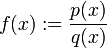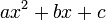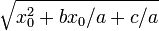# Rules for determining interval of convergence of power series of rational function

This article describes a rule, set of rules, or procedure, for determining the set of values for a parameter where a series or integral defined in terms of that parameter converges.
See other rules for determining interval of convergence

## Goal

Suppose we have a rational function:$f(x) := \frac{p(x)}{q(x)}$

Assume that$p$ and$q$ have no common divisors.

Consider a point$x_0$ such that$q(x_0) \ne 0$. Then,$f$ is locally analytic at$x_0$: the Taylor series of$f$ at$x_0$ converges to$x_0$ in an open interval centered at$x_0$. In fact, this open interval coincides with the interval of convergence of the Taylor series, i.e., the Taylor series converges to$f$ wherever it does converge.

The interval of convergence is an open interval. Below, we give the rules to determine this interval.

### Case that$q$ is a constant, so$f$ is polynomial

In this case, the interval of convergence is all of$\R$.

### Case that$q$ splits completely over$\R$

In case$q$ is expressible as a product of (possibly repeated) linear factors, the radius of convergence of the power series for$f$ is given as the minimum of the distances between$x_0$ and the zeros of$q$. Note that this minimum is positive, as$q$ has finitely many zeros, and none of them equals$x_0$ by assumption$q(x_0) \ne 0$.

The interval of convergence is the open interval centered at$x_0$ with the above radius of convergence.

### Case that$q$ has irreducible quadratic factors

Using complex number language: split$q$ completely as a product of (possibly repeated) linear factors over the complex numbers. Then, the radius of convergence of the power series for$f$ is given as the minimum of the distances between$x_0$ and the (real and non-real) zeros of$q$. Here, the distance between two complex numbers is defined as the modulus of their difference.

Using real number language: Take the minimum of the following: for each linear factor, the distance from$x_0$ to the corresponding zero, and for an irreducible quadratic factor$ax^2 + bx + c$, the value$\sqrt{x_0^2 + bx_0/a + c/a}$.

The interval of convergence is the open interval centered at$x_0$ with the above radius of convergence.